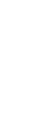# Scales of Measurement / Level of Measurement

## The Four Scales of Measurement

Watch the video for an overview of the four scales of measurement.

Watch this video on YouTube.

Data can be classified as being on one of four scales: nominal, ordinal, interval or ratio. Each level of measurement has some important properties that are useful to know. For example, only the ratio scale has meaningful zeros.A pie chart displays groups of nominal variables (i.e. categories).1. Nominal Scale. Nominal variables (also called categorical variables) can be placed into categories. They don’t have a numeric value and so cannot be added, subtracted, divided or multiplied. They also have no order; if they appear to have an order then you probably have ordinal variables instead.The ordinal scale classifies according to rank.2. Ordinal Scale. The ordinal scale contains things that you can place in order. For example, hottest to coldest, lightest to heaviest, richest to poorest. Basically, if you can rank data by 1st, 2nd, 3rd place (and so on), then you have data that’s on an ordinal scale.3. Interval Scale. An interval scale has ordered numbers with meaningful divisions. Temperature is on the interval scale: a difference of 10 degrees between 90 and 100 means the same as 10 degrees between 150 and 160. Compare that to high school ranking (which is ordinal), where the difference between 1st and 2nd might be .01 and between 10th and 11th .5. If you have meaningful divisions, you have something on the interval scale.Weight is measured on the ratio scale.4. Ratio Scale. The ratio scale is exactly the same as the interval scale with one major difference: zero is meaningful. For example, a height of zero is meaningful (it means you don’t exist). Compare that to a temperature of zero, which while it exists, it doesn’t mean anything in particular (although admittedly, in the Celsius scale it’s the freezing point for water).

## References

Agresti A. (1990) Categorical Data Analysis. John Wiley and Sons, New York.
Dodge, Y. (2008). The Concise Encyclopedia of Statistics. Springer.
Everitt, B. S.; Skrondal, A. (2010), The Cambridge Dictionary of Statistics, Cambridge University Press.

CITE THIS AS:
Stephanie Glen. "Scales of Measurement / Level of Measurement" From StatisticsHowTo.com: Elementary Statistics for the rest of us! https://www.statisticshowto.com/probability-and-statistics/descriptive-statistics/scales-of-measurement/
---------------------------------------------------------------------------Need help with a homework or test question? With Chegg Study, you can get step-by-step solutions to your questions from an expert in the field. Your first 30 minutes with a Chegg tutor is free!